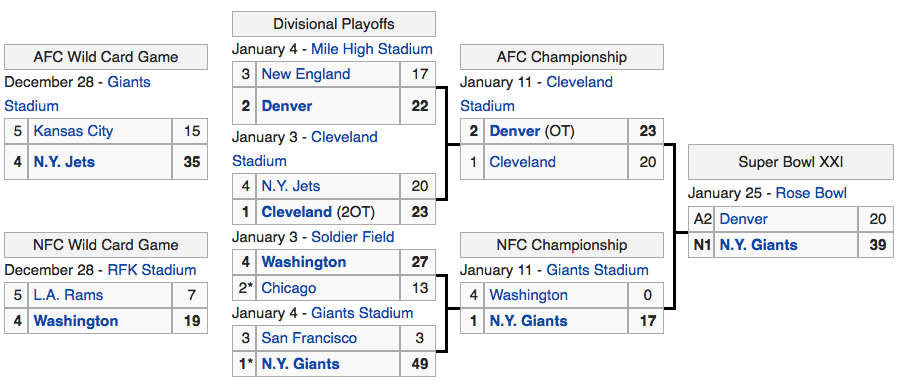## Monday, January 26, 2015

### Super Bowl 20, 21 and 22 Curiosities...Super Bowl 20Chicago = 3+8+9+3+1+7+15 = 46Super Bowl 21 NY = 14+25 = 39 New York = 5+5+5+7+6+9+2 = 39 Empire = 5+4+7+9+9+5 = 39 7/26/1788 = 7+2+6+1+7+8+8 = 39 39 Signers on Declaration of Independence 39 Books in Old Testament 39 Floors at the U.N. in New YorkSuper Bowl 22Washington is the 42nd StateThe Seahawks (Washington) defeated Denver in their 1st SB win